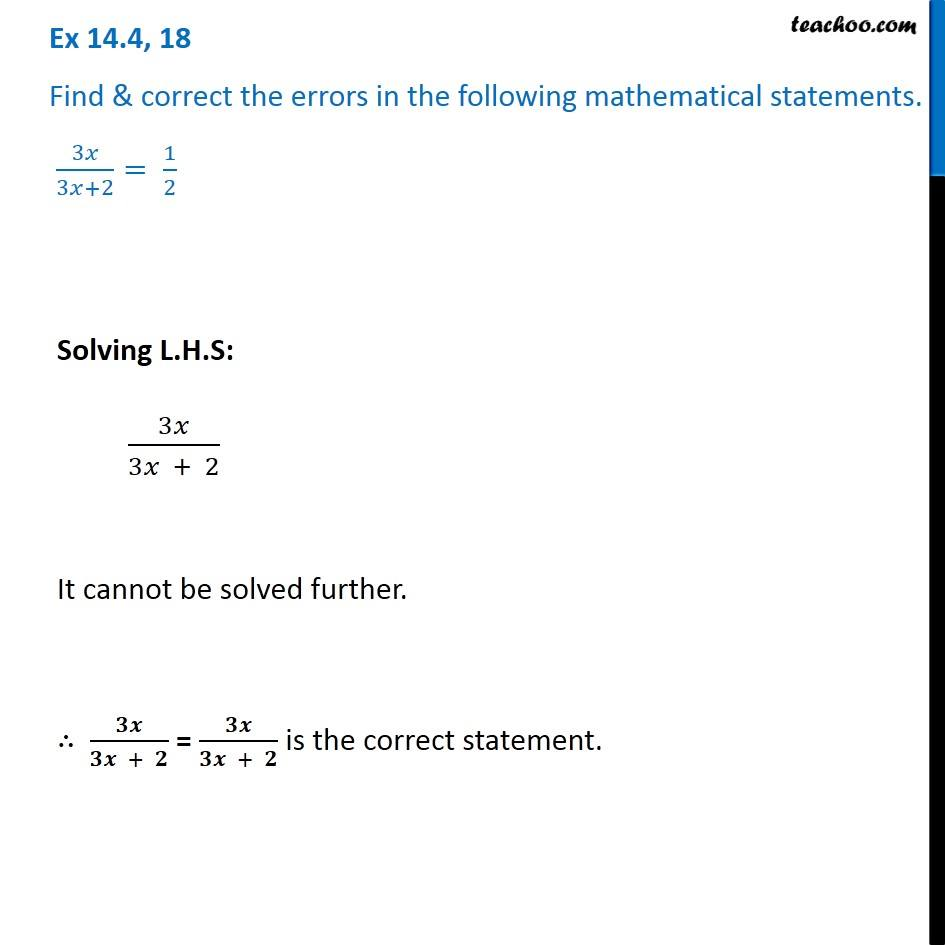1. Chapter 14 Class 8 Factorisation
2. Concept wise
3. Finding Errors

Transcript

Ex 14.4, 18 Find & correct the errors in the following mathematical statements. 3𝑥/(3𝑥+2)= 1/2 Solving L.H.S: 3𝑥/(3𝑥 + 2) It cannot be solved further. ∴ 𝟑𝒙/(𝟑𝒙 + 𝟐) = 𝟑𝒙/(𝟑𝒙 + 𝟐) is the correct statement.

Finding Errors